# Chapter Five 1 Gases Prentice Hall 2005 General

• Slides: 59Chapter Five 1 Gases Prentice Hall © 2005 General Chemistry 4 th edition, Hill, Petrucci, Mc. Creary, Perry Chapter Five2 Gases … What Are They Like? • Gases are composed of widely separated particles in constant, random motion. • Gases flow readily and occupy the entire volume of their container. • Vapor – a gas that is a liquid at room temperature and pressure (water vapor and methanol vapor, but gaseous oxygen and gaseous hydrogen). • Many low molar mass molecular compounds are either gases or easily vaporizable liquids. Prentice Hall © 2005 General Chemistry 4 th edition, Hill, Petrucci, Mc. Creary, Perry Chapter Five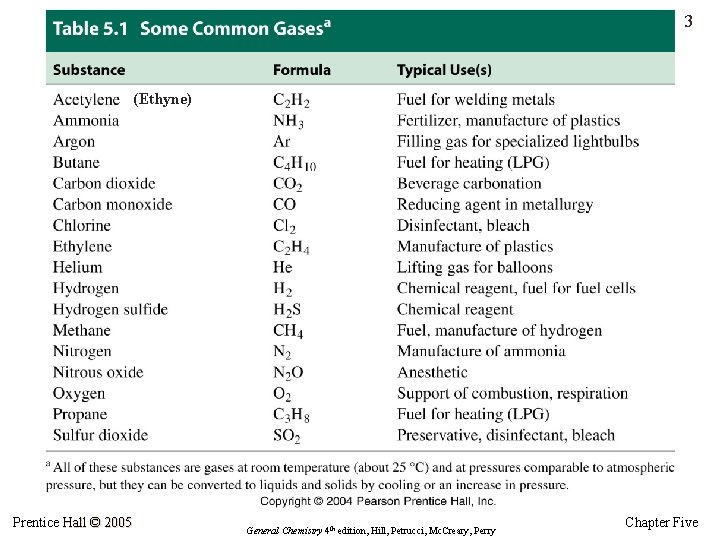3 (Ethyne) Prentice Hall © 2005 General Chemistry 4 th edition, Hill, Petrucci, Mc. Creary, Perry Chapter Five4 An Introduction to Kinetic-Molecular Theory • Provides a model for gases at the microscopic level. • Molecules are in rapid, random motion. • Movement of gases through three-dimensional space is called translational motion. • Pressure: collision of gas molecules with wall of container. • Temperature: related to average speed of gas molecules. Prentice Hall © 2005 General Chemistry 4 th edition, Hill, Petrucci, Mc. Creary, Perry Chapter Five5 Gas Pressure • Pressure is the force per unit area. • In SI, force is expressed in newtons (N) and area in square meters (m 2). • The unit of pressure in SI is the pascal (Pa) with the units N/m 2. • Kilopascals (k. Pa) are often used instead since the pascal is such a small unit. • The atmosphere and mm. Hg (Torr) are the most common scientific units for pressure. • Converting from one unit to another simply requires the appropriate conversion factor(s). Prentice Hall © 2005 General Chemistry 4 th edition, Hill, Petrucci, Mc. Creary, Perry Chapter Five6 Prentice Hall © 2005 General Chemistry 4 th edition, Hill, Petrucci, Mc. Creary, Perry Chapter Five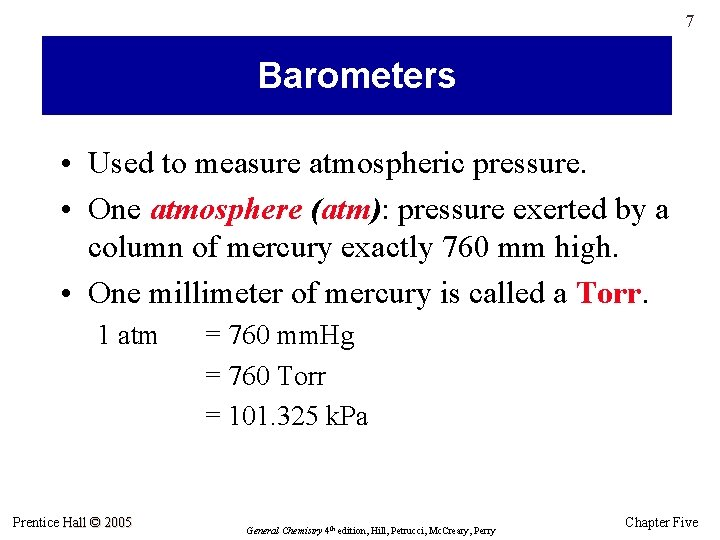7 Barometers • Used to measure atmospheric pressure. • One atmosphere (atm): pressure exerted by a column of mercury exactly 760 mm high. • One millimeter of mercury is called a Torr. 1 atm Prentice Hall © 2005 = 760 mm. Hg = 760 Torr = 101. 325 k. Pa General Chemistry 4 th edition, Hill, Petrucci, Mc. Creary, Perry Chapter Five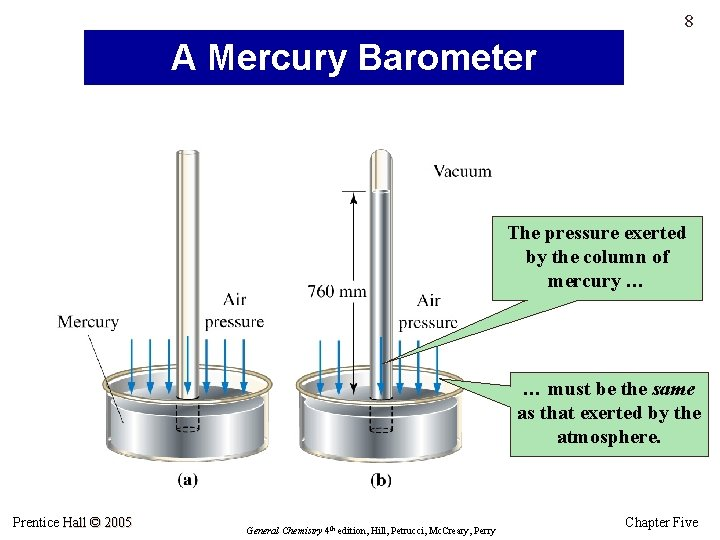8 A Mercury Barometer The pressure exerted by the column of mercury … … must be the same as that exerted by the atmosphere. Prentice Hall © 2005 General Chemistry 4 th edition, Hill, Petrucci, Mc. Creary, Perry Chapter Five9 Example 5. 1 A Canadian weather report gives the atmospheric pressure as 100. 2 k. Pa. What is the pressure expressed in the unit Torr? Prentice Hall © 2005 General Chemistry 4 th edition, Hill, Petrucci, Mc. Creary, Perry Chapter Five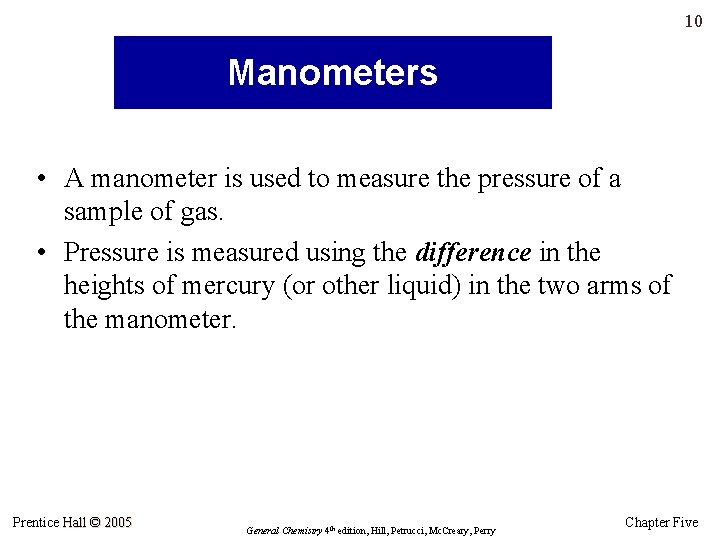10 Manometers • A manometer is used to measure the pressure of a sample of gas. • Pressure is measured using the difference in the heights of mercury (or other liquid) in the two arms of the manometer. Prentice Hall © 2005 General Chemistry 4 th edition, Hill, Petrucci, Mc. Creary, Perry Chapter Five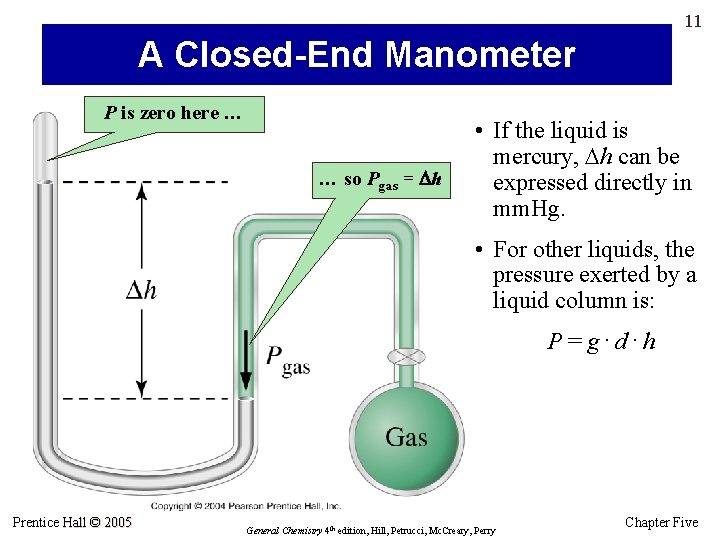11 A Closed-End Manometer P is zero here … … so Pgas = Dh • If the liquid is mercury, Dh can be expressed directly in mm. Hg. • For other liquids, the pressure exerted by a liquid column is: P=g. d. h Prentice Hall © 2005 General Chemistry 4 th edition, Hill, Petrucci, Mc. Creary, Perry Chapter Five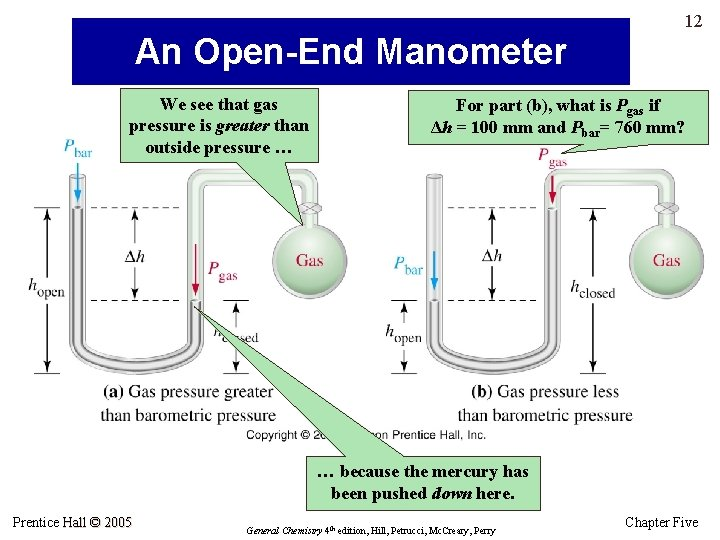12 An Open-End Manometer We see that gas pressure is greater than outside pressure … For part (b), what is Pgas if Δh = 100 mm and Pbar= 760 mm? … because the mercury has been pushed down here. Prentice Hall © 2005 General Chemistry 4 th edition, Hill, Petrucci, Mc. Creary, Perry Chapter Five13 Example 5. 2 Calculate the height of a column of water (d = 1. 00 g/cm 3) that exerts the same pressure as a column of mercury (d = 13. 6 g/cm 3) 760 mm high. Prentice Hall © 2005 General Chemistry 4 th edition, Hill, Petrucci, Mc. Creary, Perry Chapter Five14 Example 5. 3: A Conceptual Example Without doing calculations, arrange the drawings in Figure 5. 5 so that the pressures denoted in red are in increasing order. Prentice Hall © 2005 General Chemistry 4 th edition, Hill, Petrucci, Mc. Creary, Perry Chapter Five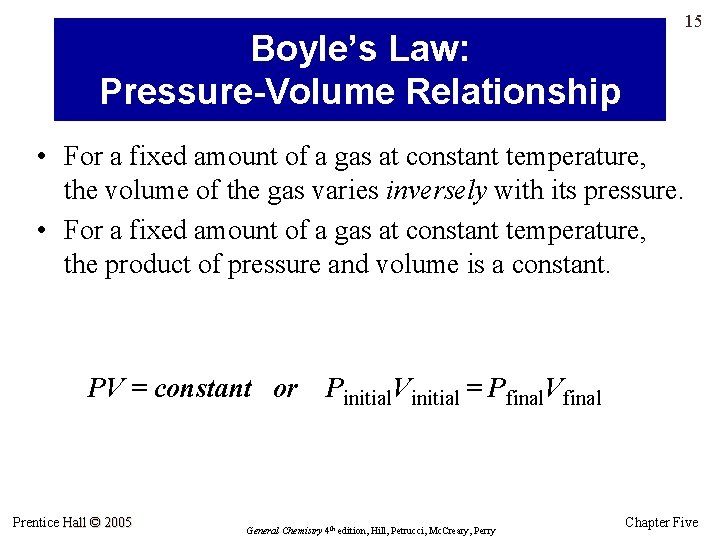15 Boyle’s Law: Pressure-Volume Relationship • For a fixed amount of a gas at constant temperature, the volume of the gas varies inversely with its pressure. • For a fixed amount of a gas at constant temperature, the product of pressure and volume is a constant. PV = constant or Prentice Hall © 2005 Pinitial. Vinitial = Pfinal. Vfinal General Chemistry 4 th edition, Hill, Petrucci, Mc. Creary, Perry Chapter Five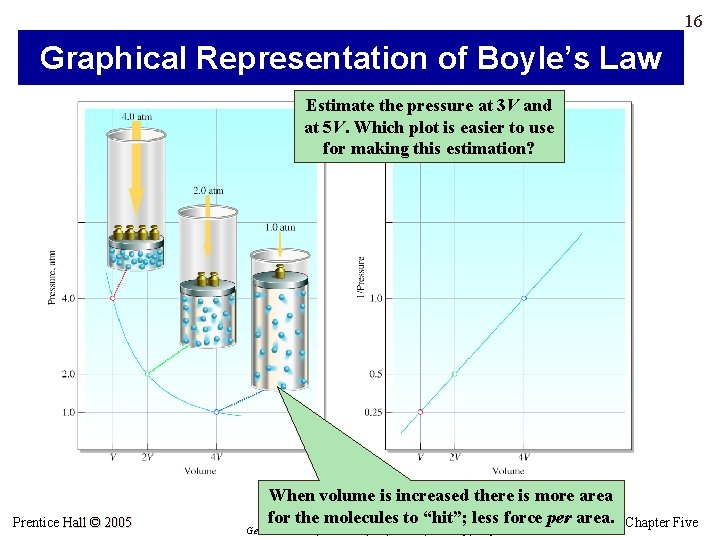16 Graphical Representation of Boyle’s Law Estimate the pressure at 3 V and at 5 V. Which plot is easier to use for making this estimation? Prentice Hall © 2005 When volume is increased there is more area for the molecules to “hit”; less force per area. General Chemistry 4 th edition, Hill, Petrucci, Mc. Creary, Perry Chapter Five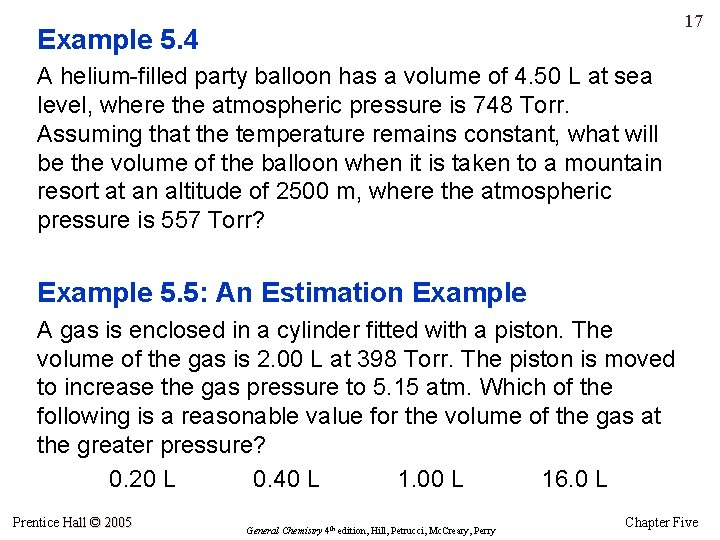17 Example 5. 4 A helium-filled party balloon has a volume of 4. 50 L at sea level, where the atmospheric pressure is 748 Torr. Assuming that the temperature remains constant, what will be the volume of the balloon when it is taken to a mountain resort at an altitude of 2500 m, where the atmospheric pressure is 557 Torr? Example 5. 5: An Estimation Example A gas is enclosed in a cylinder fitted with a piston. The volume of the gas is 2. 00 L at 398 Torr. The piston is moved to increase the gas pressure to 5. 15 atm. Which of the following is a reasonable value for the volume of the gas at the greater pressure? 0. 20 L 0. 40 L 1. 00 L 16. 0 L Prentice Hall © 2005 General Chemistry 4 th edition, Hill, Petrucci, Mc. Creary, Perry Chapter Five18 Charles’s Law: Temperature-Volume Relationship • The volume of a fixed amount of a gas at constant pressure is directly proportional to its Kelvin (absolute) temperature. • Absolute zero is the temperature obtained by extrapolation to zero volume. • Absolute zero on the Kelvin scale = – 273. 15 °C Prentice Hall © 2005 General Chemistry 4 th edition, Hill, Petrucci, Mc. Creary, Perry Chapter Five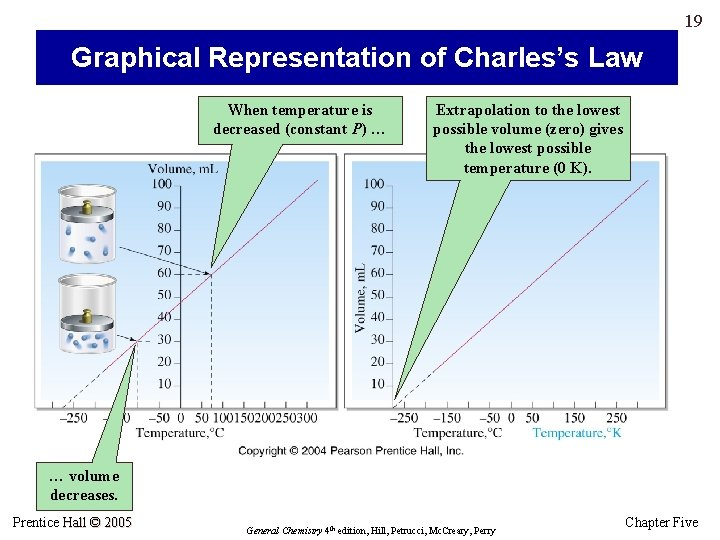19 Graphical Representation of Charles’s Law When temperature is decreased (constant P) … Extrapolation to the lowest possible volume (zero) gives the lowest possible temperature (0 K). … volume decreases. Prentice Hall © 2005 General Chemistry 4 th edition, Hill, Petrucci, Mc. Creary, Perry Chapter Five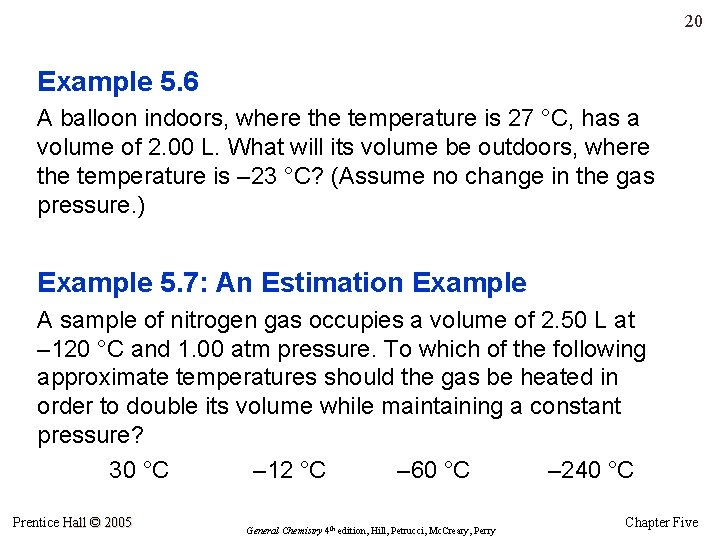20 Example 5. 6 A balloon indoors, where the temperature is 27 °C, has a volume of 2. 00 L. What will its volume be outdoors, where the temperature is – 23 °C? (Assume no change in the gas pressure. ) Example 5. 7: An Estimation Example A sample of nitrogen gas occupies a volume of 2. 50 L at – 120 °C and 1. 00 atm pressure. To which of the following approximate temperatures should the gas be heated in order to double its volume while maintaining a constant pressure? 30 °C – 12 °C – 60 °C – 240 °C Prentice Hall © 2005 General Chemistry 4 th edition, Hill, Petrucci, Mc. Creary, Perry Chapter Five21 Avogadro’s Law: Mole-Volume Relationship • At a fixed temperature and pressure, the volume of a gas is directly proportional to the amount of gas in moles (n) or to the number of molecules of gas. Van V = cn V/n = c • Standard temperature and pressure (STP) is equal to 0 °C and 1 atm. • The molar volume of a gas is the volume occupied by one mole of the gas. • At STP, molar volume of an ideal gas is 22. 4 liters. Prentice Hall © 2005 General Chemistry 4 th edition, Hill, Petrucci, Mc. Creary, Perry Chapter Five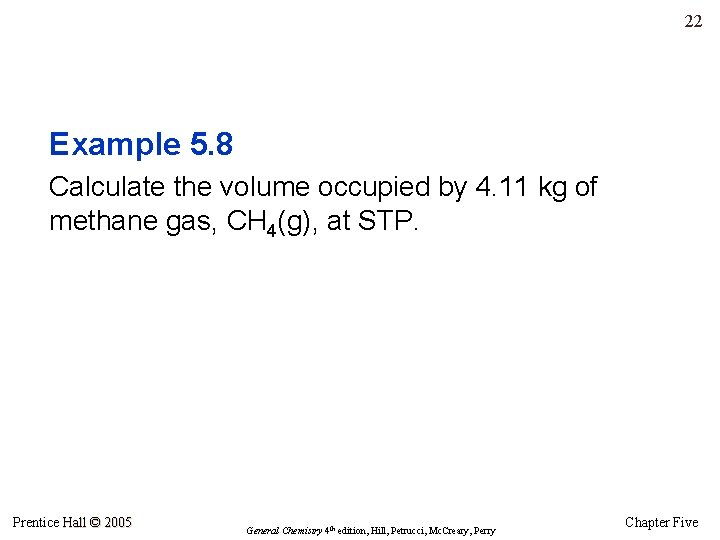22 Example 5. 8 Calculate the volume occupied by 4. 11 kg of methane gas, CH 4(g), at STP. Prentice Hall © 2005 General Chemistry 4 th edition, Hill, Petrucci, Mc. Creary, Perry Chapter Five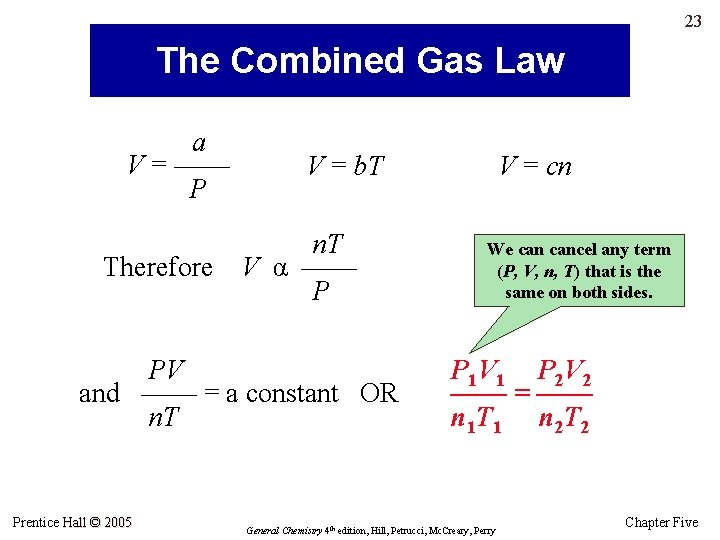23 The Combined Gas Law a V = —— P Therefore V = b. T n. T V α —— P PV and —— = a constant OR n. T Prentice Hall © 2005 V = cn We cancel any term (P, V, n, T) that is the same on both sides. P 1 V 1 P 2 V 2 —— = —— n 1 T 1 n 2 T 2 General Chemistry 4 th edition, Hill, Petrucci, Mc. Creary, Perry Chapter Five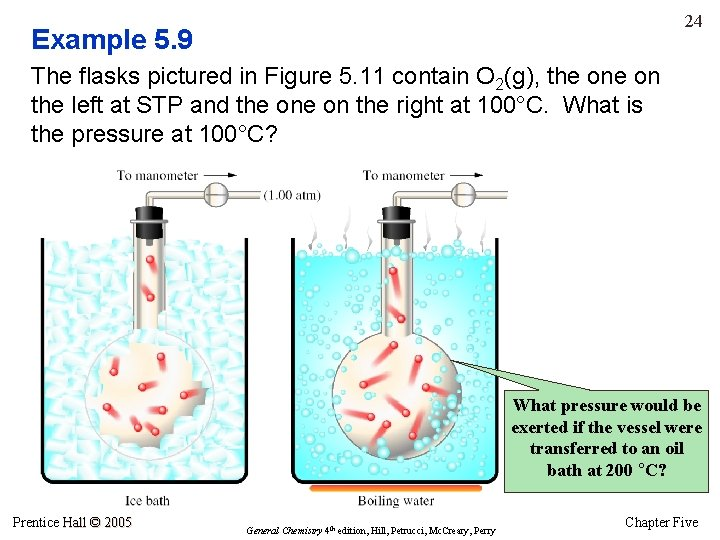24 Example 5. 9 The flasks pictured in Figure 5. 11 contain O 2(g), the on the left at STP and the on the right at 100°C. What is the pressure at 100°C? What pressure would be exerted if the vessel were transferred to an oil bath at 200 °C? Prentice Hall © 2005 General Chemistry 4 th edition, Hill, Petrucci, Mc. Creary, Perry Chapter Five25 The Ideal Gas Law PV —— = constant = R n. T PV = n. RT R = 0. 08206 (L·atm)/(mol·K) The ideal gas constant The ideal gas law • P in atm, V in L, n in moles, T in kelvins. • If any other units are used for these variables, a different value for R must be used … Prentice Hall © 2005 General Chemistry 4 th edition, Hill, Petrucci, Mc. Creary, Perry Chapter Five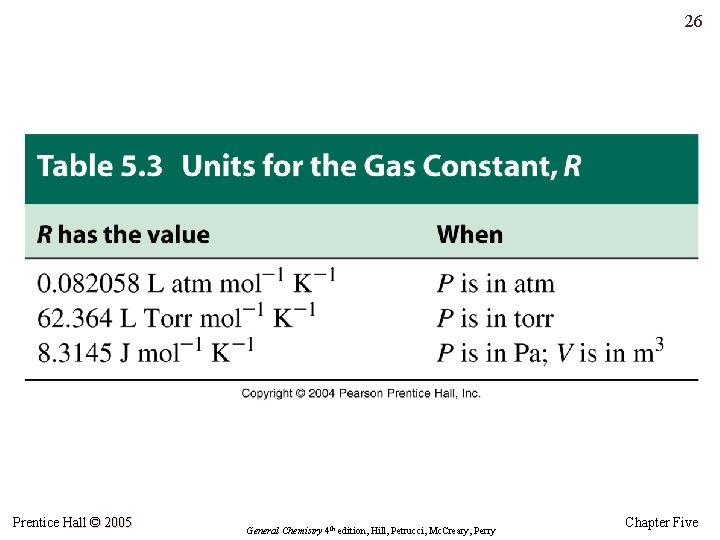26 Prentice Hall © 2005 General Chemistry 4 th edition, Hill, Petrucci, Mc. Creary, Perry Chapter Five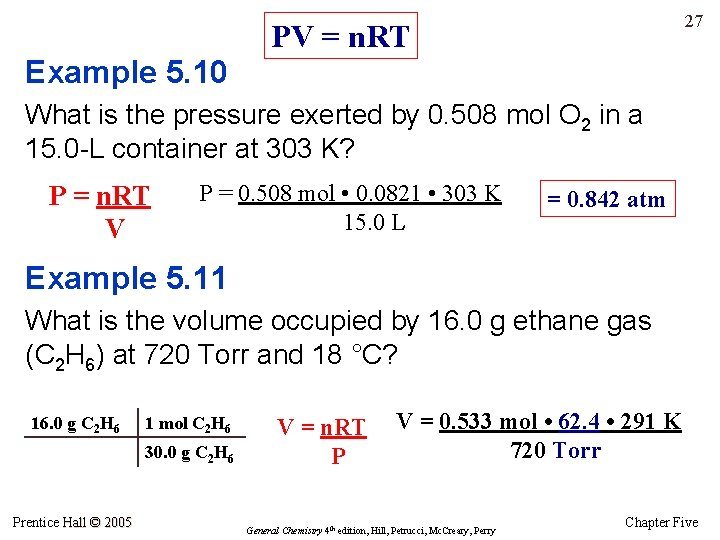27 PV = n. RT Example 5. 10 What is the pressure exerted by 0. 508 mol O 2 in a 15. 0 -L container at 303 K? P = n. RT V P = 0. 508 mol • 0. 0821 • 303 K 15. 0 L = 0. 842 atm Example 5. 11 What is the volume occupied by 16. 0 g ethane gas (C 2 H 6) at 720 Torr and 18 °C? 16. 0 g C 2 H 6 1 mol C 2 H 6 30. 0 g C 2 H 6 Prentice Hall © 2005 V = n. RT P V = 0. 533 mol • 62. 4 • 291 K 720 Torr General Chemistry 4 th edition, Hill, Petrucci, Mc. Creary, Perry Chapter FiveApplications of the Ideal Gas Law: Molecular Mass Determination 28 M = molar mass and m = mass in grams m (grams) M = ————— n (moles) m n= — M so PV n = —— RT The ideal gas equation rearranges to: Setting the equations equal to one another: m. RT … and solving for M: M = ——— PV Prentice Hall © 2005 PV — = —— M RT m Alternative to equation: (A) find n using the ideal gas equation; (B) Divide m (grams) by n (moles) to get grams/mol. General Chemistry 4 th edition, Hill, Petrucci, Mc. Creary, Perry Chapter Five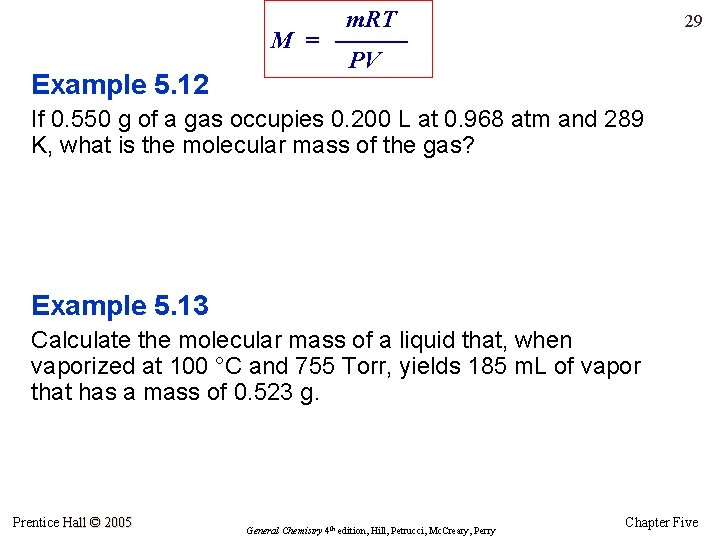Example 5. 12 m. RT M = ——— PV 29 If 0. 550 g of a gas occupies 0. 200 L at 0. 968 atm and 289 K, what is the molecular mass of the gas? Example 5. 13 Calculate the molecular mass of a liquid that, when vaporized at 100 °C and 755 Torr, yields 185 m. L of vapor that has a mass of 0. 523 g. Prentice Hall © 2005 General Chemistry 4 th edition, Hill, Petrucci, Mc. Creary, Perry Chapter Five30 Applications of the Ideal Gas Law: Gas Densities • Gases are much less dense than liquids and solids, so gas densities are usually reported in g/L. m. RT M = ——— PV rearranges to m and density = — V so m MP — = —— V RT MP d = —— RT Alternative: find volume of one mole (n = 1) or other fixed quantity of gas. Divide mass of that quantity by volume to find g/L. Density of a gas is directly proportional to its molar mass and pressure, and is inversely proportional to Kelvin temperature. Prentice Hall © 2005 General Chemistry 4 th edition, Hill, Petrucci, Mc. Creary, Perry Chapter Five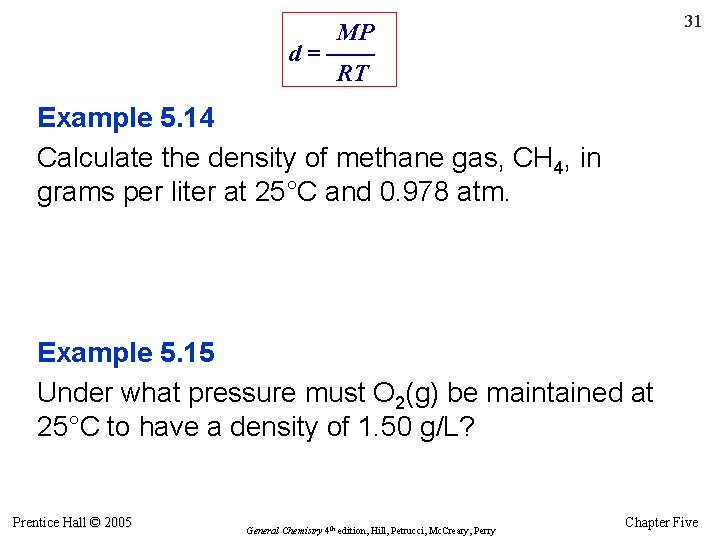31 MP d = —— RT Example 5. 14 Calculate the density of methane gas, CH 4, in grams per liter at 25°C and 0. 978 atm. Example 5. 15 Under what pressure must O 2(g) be maintained at 25°C to have a density of 1. 50 g/L? Prentice Hall © 2005 General Chemistry 4 th edition, Hill, Petrucci, Mc. Creary, Perry Chapter Five32 Gases in Reaction Stoichiometry: The Law of Combining Volumes • When gases measured at the same temperature and pressure allowed to react, the volumes of gaseous reactants and products are in small whole-number ratios. • Example: At a given temperature and pressure, 2. 00 L of H 2 will react with 1. 00 L of O 2 (Why 2: 1? Balance the equation …) • Example: At a given temperature and pressure, 6. 00 L of H 2 of will react with 2. 00 L of N 2 to form 4. 00 L of NH 3 (Why 6: 2: 4? Balance the equation …) • We don’t need to know actual conditions for the reaction … as long as the same conditions apply to all the gases. Prentice Hall © 2005 General Chemistry 4 th edition, Hill, Petrucci, Mc. Creary, Perry Chapter Five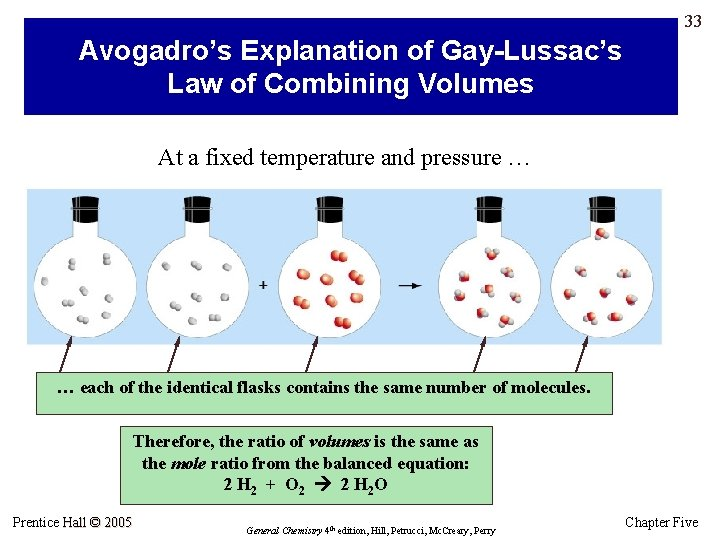33 Avogadro’s Explanation of Gay-Lussac’s Law of Combining Volumes At a fixed temperature and pressure … … each of the identical flasks contains the same number of molecules. Therefore, the ratio of volumes is the same as the mole ratio from the balanced equation: 2 H 2 + O 2 2 H 2 O Prentice Hall © 2005 General Chemistry 4 th edition, Hill, Petrucci, Mc. Creary, Perry Chapter Five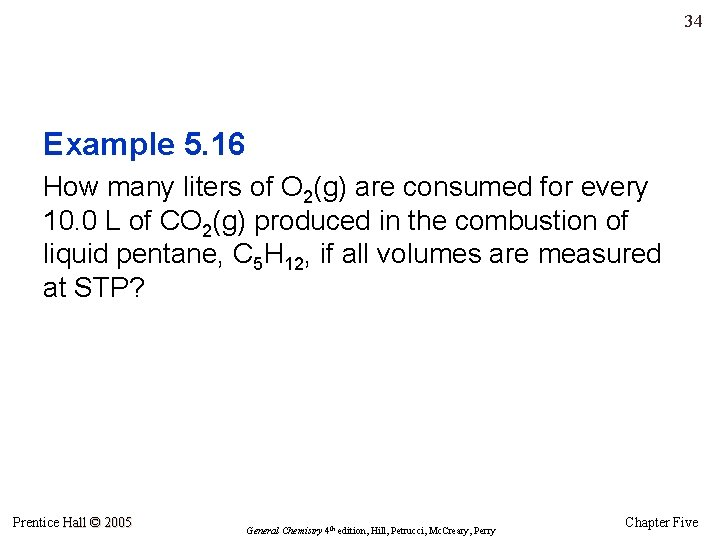34 Example 5. 16 How many liters of O 2(g) are consumed for every 10. 0 L of CO 2(g) produced in the combustion of liquid pentane, C 5 H 12, if all volumes are measured at STP? Prentice Hall © 2005 General Chemistry 4 th edition, Hill, Petrucci, Mc. Creary, Perry Chapter Five35 The Ideal Gas Equation in Reaction Stoichiometry • We can use the law of combining volumes for stoichiometry only for gases and only if the gases are at the same temperature and pressure. • Otherwise, we must use stoichiometric methods from Chapter 3 – combined with the ideal gas equation. Prentice Hall © 2005 General Chemistry 4 th edition, Hill, Petrucci, Mc. Creary, Perry Chapter Five36 The Ideal Gas Equation in Reaction Stoichiometry • As in other stoichiometry calculations, the problem centers around the mole ratio: Remember this? From Chapter 3, Stoichiometry? • If A is a gas, we find moles of A first by using the ideal gas equation and P, V, and T. • If B is a gas, we solve for moles of B (n), then use the ideal gas equation to find P, V, or T. Prentice Hall © 2005 General Chemistry 4 th edition, Hill, Petrucci, Mc. Creary, Perry Chapter Five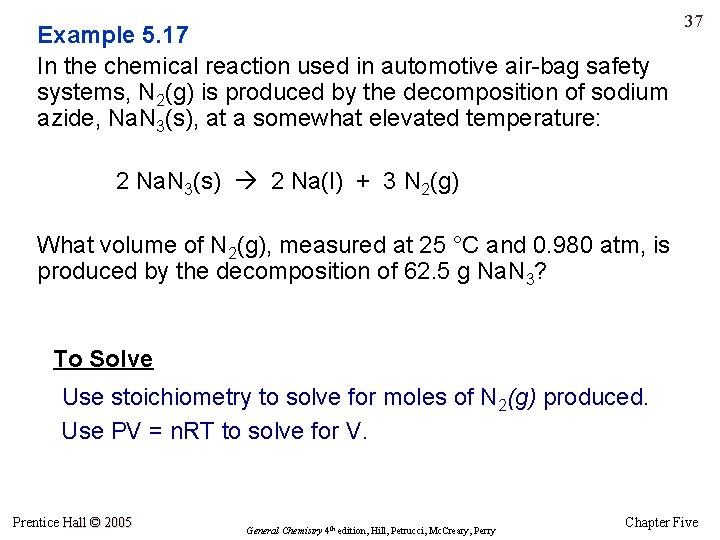Example 5. 17 In the chemical reaction used in automotive air-bag safety systems, N 2(g) is produced by the decomposition of sodium azide, Na. N 3(s), at a somewhat elevated temperature: 37 2 Na. N 3(s) 2 Na(l) + 3 N 2(g) What volume of N 2(g), measured at 25 °C and 0. 980 atm, is produced by the decomposition of 62. 5 g Na. N 3? To Solve Use stoichiometry to solve for moles of N 2(g) produced. Use PV = n. RT to solve for V. Prentice Hall © 2005 General Chemistry 4 th edition, Hill, Petrucci, Mc. Creary, Perry Chapter FiveMixtures of Gases: Dalton’s Law of Partial Pressures 38 • Dalton’s law of partial pressures is used in dealing with mixtures of gases. • The total pressure exerted by a mixture of gases is equal to the sum of the partial pressures exerted by the separate gases: Ptotal = P 1 + P 2 + P 3 + … Partial pressure: the pressure a gas would exert if it were alone in the container. n 1 RT P 1 = ——— V Prentice Hall © 2005 n 2 RT P 2 = ——— V n 3 RT P 3 = ——— … V General Chemistry 4 th edition, Hill, Petrucci, Mc. Creary, Perry Chapter Five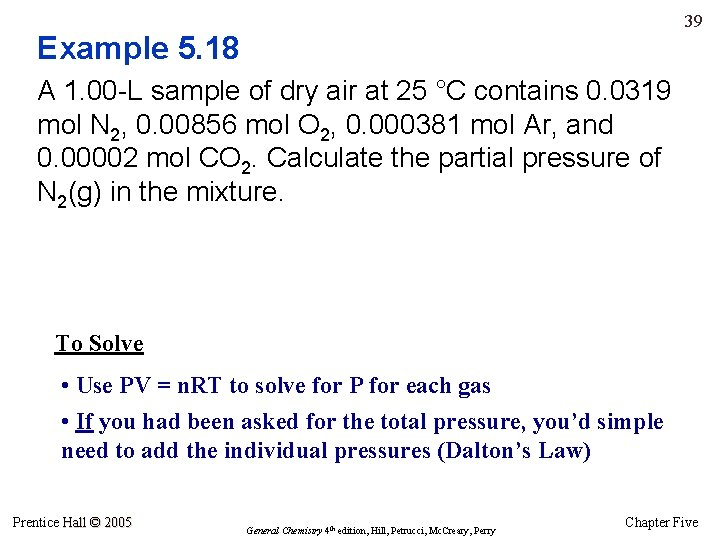39 Example 5. 18 A 1. 00 -L sample of dry air at 25 °C contains 0. 0319 mol N 2, 0. 00856 mol O 2, 0. 000381 mol Ar, and 0. 00002 mol CO 2. Calculate the partial pressure of N 2(g) in the mixture. To Solve • Use PV = n. RT to solve for P for each gas • If you had been asked for the total pressure, you’d simple need to add the individual pressures (Dalton’s Law) Prentice Hall © 2005 General Chemistry 4 th edition, Hill, Petrucci, Mc. Creary, Perry Chapter FivePartial Pressures Illustrated 40 What would be the total pressure if 0. 90 mol of N 2 were added to the gas mixture in (c)? Partial pressure of H 2 is 2. 9 atm. Partial pressure of He is 7. 2 atm. Hmm … partial pressure appears to be related to the number of moles of gas … Prentice Hall © 2005 General Chemistry 4 th edition, Hill, Petrucci, Mc. Creary, Perry Chapter Five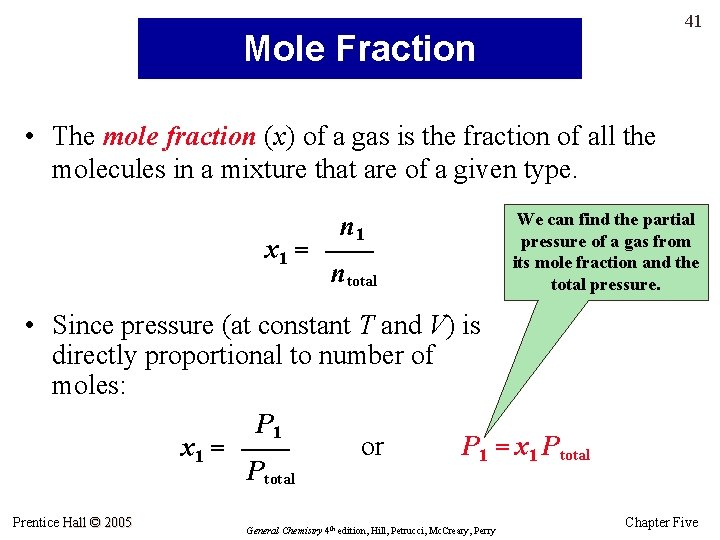41 Mole Fraction • The mole fraction (x) of a gas is the fraction of all the molecules in a mixture that are of a given type. n 1 x 1 = —— ntotal We can find the partial pressure of a gas from its mole fraction and the total pressure. • Since pressure (at constant T and V) is directly proportional to number of moles: P 1 or P 1 = x 1 Ptotal x 1 = —— Ptotal Prentice Hall © 2005 General Chemistry 4 th edition, Hill, Petrucci, Mc. Creary, Perry Chapter Five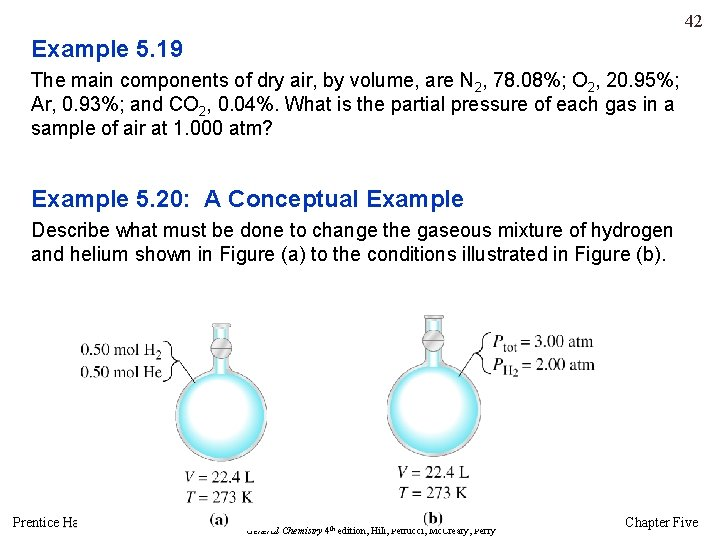42 Example 5. 19 The main components of dry air, by volume, are N 2, 78. 08%; O 2, 20. 95%; Ar, 0. 93%; and CO 2, 0. 04%. What is the partial pressure of each gas in a sample of air at 1. 000 atm? Example 5. 20: A Conceptual Example Describe what must be done to change the gaseous mixture of hydrogen and helium shown in Figure (a) to the conditions illustrated in Figure (b). Prentice Hall © 2005 General Chemistry 4 th edition, Hill, Petrucci, Mc. Creary, Perry Chapter Five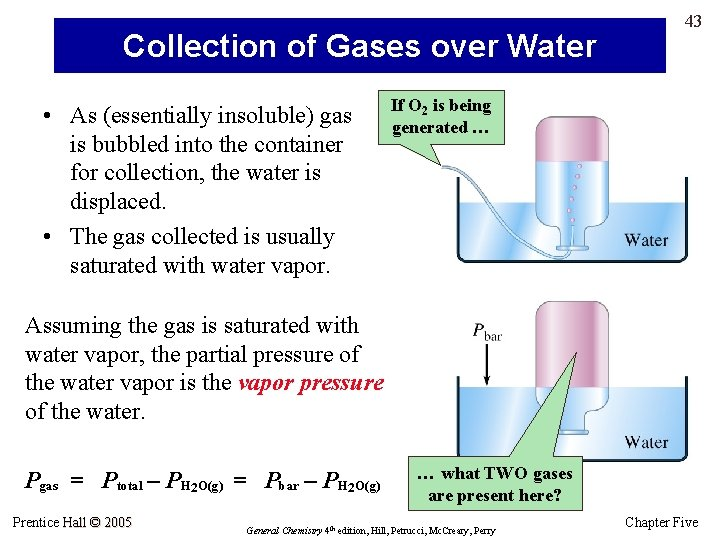Collection of Gases over Water • As (essentially insoluble) gas is bubbled into the container for collection, the water is displaced. • The gas collected is usually saturated with water vapor. 43 If O 2 is being generated … Assuming the gas is saturated with water vapor, the partial pressure of the water vapor is the vapor pressure of the water. Pgas = Ptotal – PH 2 O(g) = Pbar – PH 2 O(g) Prentice Hall © 2005 … what TWO gases are present here? General Chemistry 4 th edition, Hill, Petrucci, Mc. Creary, Perry Chapter FiveExample 5. 21 Hydrogen produced in the following reaction is collected over water at 23°C when the barometric pressure is 742 Torr: 44 2 Al(s) + 6 HCl(aq) 2 Al. Cl 3(aq) + 3 H 2(g) What volume of the “wet” gas will be collected in the reaction of 1. 50 g Al(s) with excess HCl(aq)? To Solve • The pressure given is pressure of H 2 produced plus vapor pressure of water at 23°C. Find the vapor pressure of water at 23°C (from a data table) and subtract it from the total pressure (742 Torr) to give the partial pressure of H 2 in the “wet” mixture of gases. • Use the pressure values of H 2 and H 2 O(g) to calculate a mole fraction for the gases (see previous slide - #41) • With the given mass of Al(s), calculate the moles of H 2 that will be produced • With the mole fraction, and the number of moles of H 2, you can calculate the number of moles of H 2 O(g) in the mixture. • Add moles of H 2 + H 2 O(g) = total moles of gas. Plug this n into PV = n. RT. Prentice Hall © 2005 General Chemistry 4 th edition, Hill, Petrucci, Mc. Creary, Perry Chapter Five45 The Kinetic-Molecular Theory: Some Quantitative Aspects The principal assumptions of kinetic-molecular theory are: – A gas is made up of molecules that are in constant, random, straight-line motion. – Molecules of a gas are far apart; a gas is mostly empty space. – There are no forces between molecules except during the instant of collision. – Individual molecules may gain or lose energy as a result of collisions; however, the total energy remains constant. Prentice Hall © 2005 General Chemistry 4 th edition, Hill, Petrucci, Mc. Creary, Perry Chapter Five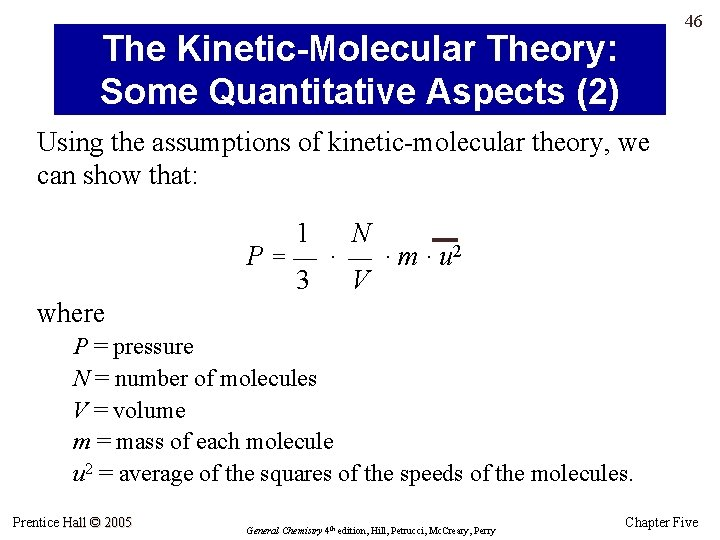46 The Kinetic-Molecular Theory: Some Quantitative Aspects (2) Using the assumptions of kinetic-molecular theory, we can show that: 1 N P = — · m · u 2 3 V where P = pressure N = number of molecules V = volume m = mass of each molecule u 2 = average of the squares of the speeds of the molecules. Prentice Hall © 2005 General Chemistry 4 th edition, Hill, Petrucci, Mc. Creary, Perry Chapter Five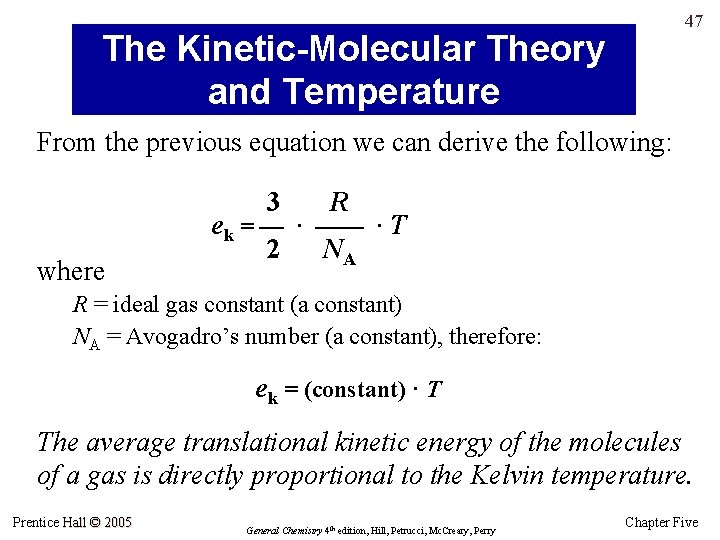47 The Kinetic-Molecular Theory and Temperature From the previous equation we can derive the following: 3 where R ek = — · —— · T 2 NA R = ideal gas constant (a constant) NA = Avogadro’s number (a constant), therefore: ek = (constant) · T The average translational kinetic energy of the molecules of a gas is directly proportional to the Kelvin temperature. Prentice Hall © 2005 General Chemistry 4 th edition, Hill, Petrucci, Mc. Creary, Perry Chapter Five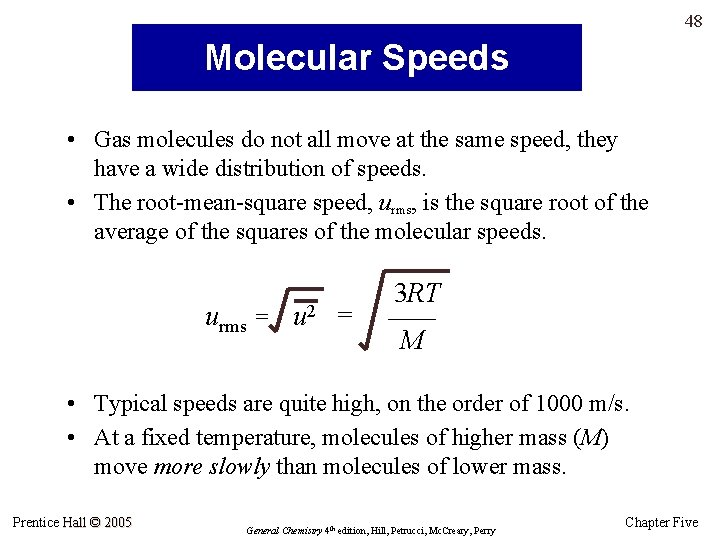48 Molecular Speeds • Gas molecules do not all move at the same speed, they have a wide distribution of speeds. • The root-mean-square speed, urms, is the square root of the average of the squares of the molecular speeds. urms = u 2 = 3 RT —— M • Typical speeds are quite high, on the order of 1000 m/s. • At a fixed temperature, molecules of higher mass (M) move more slowly than molecules of lower mass. Prentice Hall © 2005 General Chemistry 4 th edition, Hill, Petrucci, Mc. Creary, Perry Chapter Five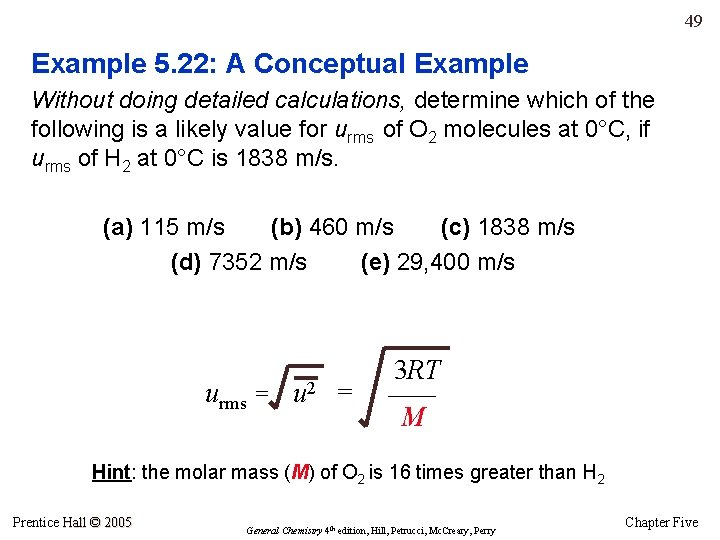49 Example 5. 22: A Conceptual Example Without doing detailed calculations, determine which of the following is a likely value for urms of O 2 molecules at 0°C, if urms of H 2 at 0°C is 1838 m/s. (a) 115 m/s (b) 460 m/s (c) 1838 m/s (d) 7352 m/s (e) 29, 400 m/s urms = u 2 = 3 RT —— M Hint: the molar mass (M) of O 2 is 16 times greater than H 2 Prentice Hall © 2005 General Chemistry 4 th edition, Hill, Petrucci, Mc. Creary, Perry Chapter Five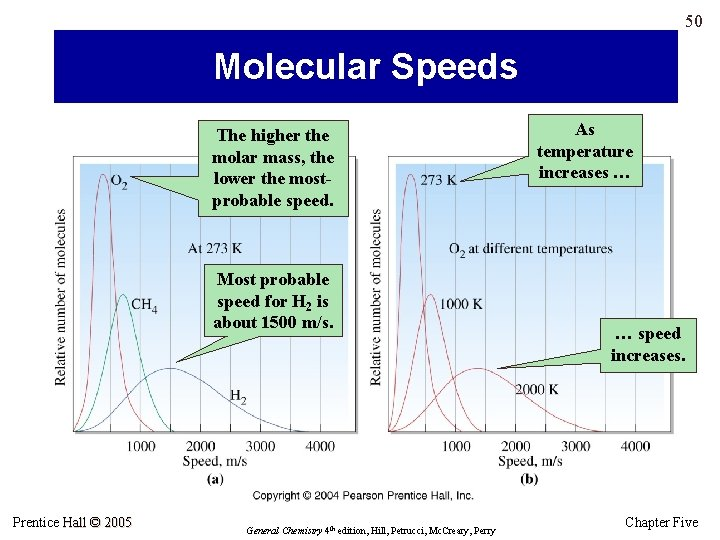50 Molecular Speeds The higher the molar mass, the lower the mostprobable speed. Most probable speed for H 2 is about 1500 m/s. Prentice Hall © 2005 General Chemistry 4 th edition, Hill, Petrucci, Mc. Creary, Perry As temperature increases … … speed increases. Chapter Five51 Diffusion • Diffusion is the movements of molecules from areas of higher to areas of lower concentration – the process by which one substance mixes with one or more other substances as a result of the random motions of molecules. – Mathematical basis – Diffusion of gases is slower than would be predicted by molecular speeds due to the frequent collisions of molecules. Prentice Hall © 2005 General Chemistry 4 th edition, Hill, Petrucci, Mc. Creary, Perry Chapter Five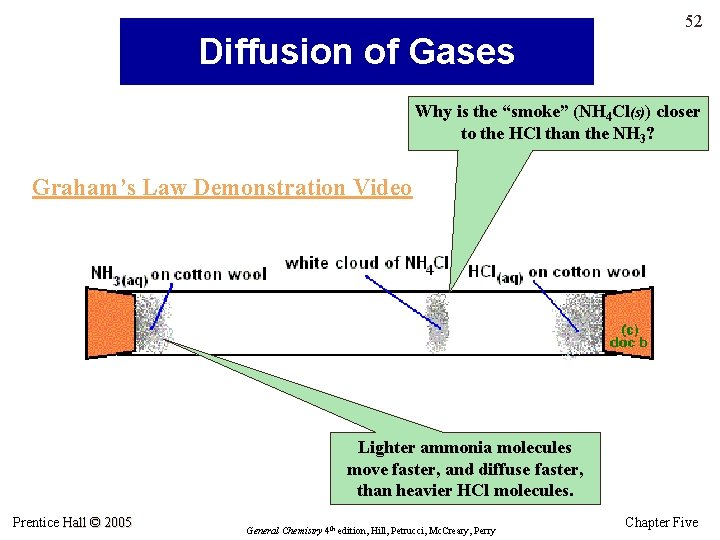52 Diffusion of Gases Why is the “smoke” (NH 4 Cl(s)) closer to the HCl than the NH 3? Graham’s Law Demonstration Video Lighter ammonia molecules move faster, and diffuse faster, than heavier HCl molecules. Prentice Hall © 2005 General Chemistry 4 th edition, Hill, Petrucci, Mc. Creary, Perry Chapter Five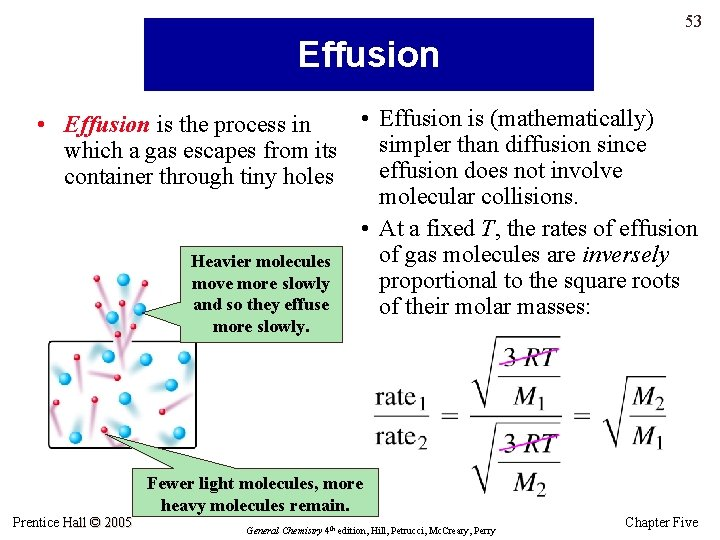53 Effusion • Effusion is (mathematically) • Effusion is the process in simpler than diffusion since which a gas escapes from its effusion does not involve container through tiny holes molecular collisions. • At a fixed T, the rates of effusion of gas molecules are inversely Heavier molecules proportional to the square roots move more slowly and so they effuse of their molar masses: more slowly. Fewer light molecules, more heavy molecules remain. Prentice Hall © 2005 General Chemistry 4 th edition, Hill, Petrucci, Mc. Creary, Perry Chapter Five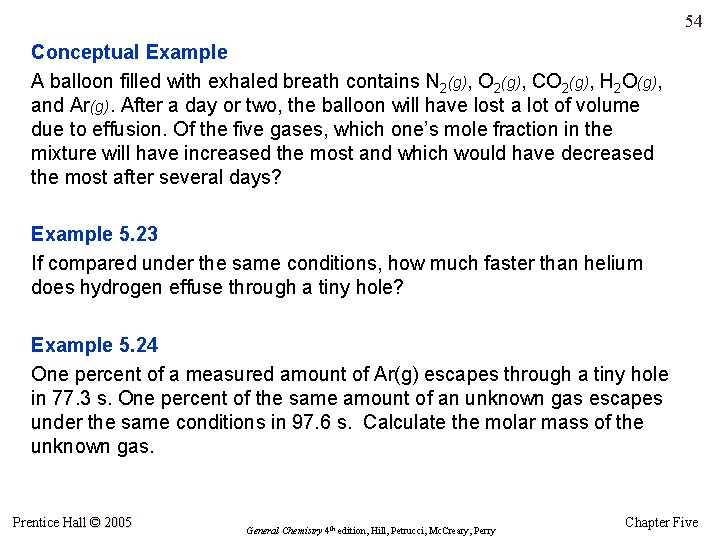54 Conceptual Example A balloon filled with exhaled breath contains N 2(g), O 2(g), CO 2(g), H 2 O(g), and Ar(g). After a day or two, the balloon will have lost a lot of volume due to effusion. Of the five gases, which one’s mole fraction in the mixture will have increased the most and which would have decreased the most after several days? Example 5. 23 If compared under the same conditions, how much faster than helium does hydrogen effuse through a tiny hole? Example 5. 24 One percent of a measured amount of Ar(g) escapes through a tiny hole in 77. 3 s. One percent of the same amount of an unknown gas escapes under the same conditions in 97. 6 s. Calculate the molar mass of the unknown gas. Prentice Hall © 2005 General Chemistry 4 th edition, Hill, Petrucci, Mc. Creary, Perry Chapter Five55 Real Gases Under some conditions, real gases do not follow the ideal gas law. 1. Intermolecular forces of attraction cause the measured pressure of a real gas to be less than expected. 2. When molecules are close together, the volume of the molecules themselves becomes a significant fraction of the total volume of a gas. Under what conditions of temperature and pressure will #1 and #2 become important? Prentice Hall © 2005 General Chemistry 4 th edition, Hill, Petrucci, Mc. Creary, Perry Chapter Five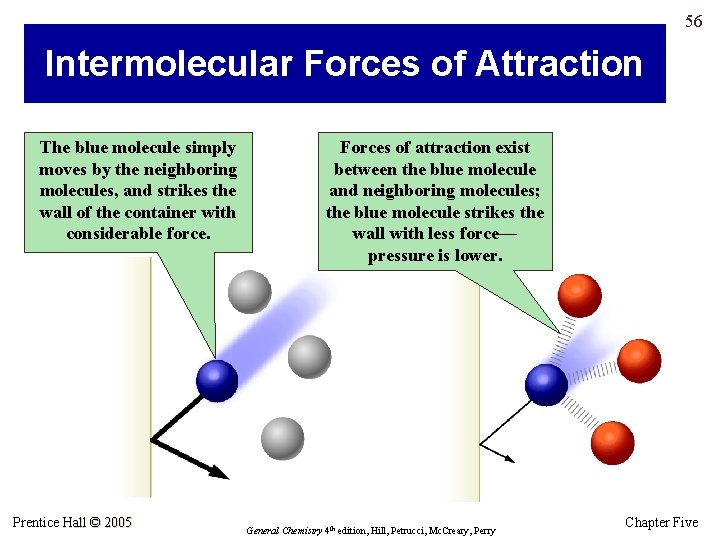56 Intermolecular Forces of Attraction The blue molecule simply moves by the neighboring molecules, and strikes the wall of the container with considerable force. Prentice Hall © 2005 Forces of attraction exist between the blue molecule and neighboring molecules; the blue molecule strikes the wall with less force— pressure is lower. General Chemistry 4 th edition, Hill, Petrucci, Mc. Creary, Perry Chapter Five57 Real Gases • • • Ideal gas equation (ideal gases): [P ] (V ) = n. RT van der Waals equation (real gases): [P + {(n 2 a)/V 2}] (V – nb) = n. RT a – term is related to intermolecular force strength. b – term is related to volume of the gas molecules (in liters per mole). Both a and b are empirical constants, determined by experiment. Prentice Hall © 2005 General Chemistry 4 th edition, Hill, Petrucci, Mc. Creary, Perry Chapter Five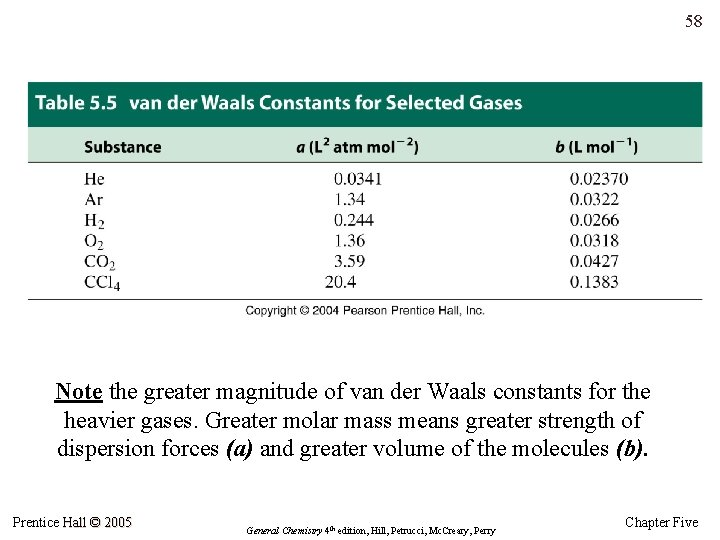58 Note the greater magnitude of van der Waals constants for the heavier gases. Greater molar mass means greater strength of dispersion forces (a) and greater volume of the molecules (b). Prentice Hall © 2005 General Chemistry 4 th edition, Hill, Petrucci, Mc. Creary, Perry Chapter Five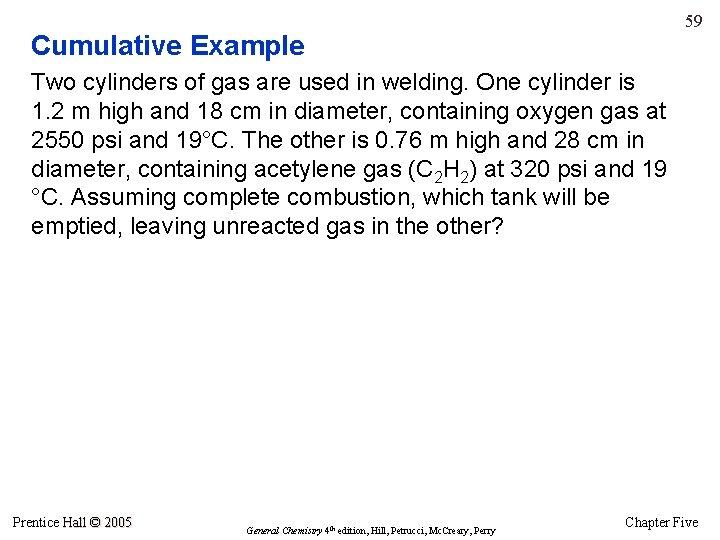59 Cumulative Example Two cylinders of gas are used in welding. One cylinder is 1. 2 m high and 18 cm in diameter, containing oxygen gas at 2550 psi and 19°C. The other is 0. 76 m high and 28 cm in diameter, containing acetylene gas (C 2 H 2) at 320 psi and 19 °C. Assuming complete combustion, which tank will be emptied, leaving unreacted gas in the other? Prentice Hall © 2005 General Chemistry 4 th edition, Hill, Petrucci, Mc. Creary, Perry Chapter Five